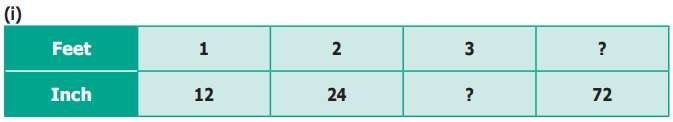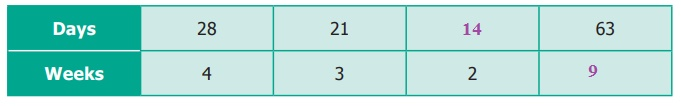Home | | Maths 6th Std | Exercise 3.2

# Exercise 3.2

6th Maths : Term 1 Unit 3 : Ratio and Proportion : Exercise 3.2 : Text Book Back Exercises Questions with Answers, Solution

Exercise 3.2

1. Fill in the blanks of the given equivalent ratios.

(i) 3 : 5 = 9 : ___

(ii) 4 : 5 = ___ : 10

(iii) 6 : ___ = 1 : 2

(i) 3 : 5 = 9 : 15

(ii) 4 : 5 = 8 : 10

(iii) 6 : 12 = 1 : 2

2. Complete the table.3. Say True or False.

(i) 5 : 7 is equivalent to 21 : 15 [False]

(ii) If 40 is divided in the ratio 3 : 2, then the larger part is 24. [True]

4. Give two equivalent ratios for each of the following.

(i) 3 : 2

(ii) 1 : 6

(iii) 5 : 4

Solution:

(i) 3:2

Fractional for = 3 / 2

Equivalent ratio 3/2 × 2/2  = 6/4     3/2 × 3/3 = 9/6

Equivalent ratios for 3 : 2 are 6 : 4, 9 : 6

(ii) 1 : 6

Fractional for = 1/6

Equivalent ratio 1/6 × 2/2 = 2/12         1/6 × 3/3 = 3/18

Equivalent ratios for 1 : 6 are 2 : 12, 3 : 18

(ii) 5 : 4

Fractional for = 5/4

Equivalent ratio = 5/4 × 2/2 = 10 /8     5/4 × 3/3 = 15/12

Equivalent ratios for 5 : 4 are 10 : 8, 15 : 12

5. Which of the two ratios is larger?

(i) 4 : 5 or 8 : 15

(ii) 3 : 4 or 7 : 8

(iii) 1 : 2 or 2 : 1

(i) 4 : 5 (or) 8 : 15

4/5 × 2/2 = 8/10           8 /15 × 2/2 = 16/30

4/5 × 3/3 = 12/15          8/15 × 3/3 = 24/30

Compaing the equivalent ratios :

12/15 × 8/15

12/15 > 8/15

We conclude that 4/5 is greater.

i.e. 4: 5 greater than 8:15

(ii) 3:4 (or) 7:8

3/4 × 2/2 = 6/8         7/ 8 × 2/2 = 14/16

Compaing the equivalent ratios : 6/8, 7/8 = 6/8 < 7/8

We conclude that 7: 8 is greater than 3:4

(iii) 1:2 (or) 2:1

1/2  × 2/2 =  2/4            2/1 × 2/2 = 4/2

Compaing the equivalent ratios : 1/2, 4/2 = 1/2 < 4/2

We conclude that 2:1 is greater than 1:2

6. Divide the numbers given below in the required ratio.

(i) 20 in the ratio 3 : 2

(ii) 27 in the ratio 4 : 5

(iii) 40 in the ratio 6 : 14

Solution:

Total parts = 3 + 2 = 5

3 parts ⇒ 20 × 3/5 = 12

2 parts ⇒ 20 × 2/5 = 8

20 in the ratio 3:2 is 12 : 8

(ii) 27 in the ratio 4: 5

Solution: Total parts = 4 + 5 = 9

4 parts  27 × 4/9 = 12

5 parts  27 × 5/9 = 15

27 in the ratio 4 : 5 is 12 : 15

(iii) 40 in the ratio 6:14

Solution: Total parts = 6 + 14 = 20

6 parts ⇒ 40 × 6/20 = 12

14 parts ⇒  40 × 14/20 = 28

7. In a family, the amount spent in a month for buying Provisions and Vegetables are in the ratio 3 : 2. If the allotted amount is ₹4000, then what will be the amount spent for (i) Provisions and (ii) Vegetables?

Solution:

Total amount = Rs.4000

Provisions : Vegetables = 3:2

Total parts = 5

(i) Provisions ⇒  4000 × 3/5 = 2400

(ii) Vegetables ⇒  4000 × 2/5 = 1600

Amount spent for Provisions = Rs.2400

Vegetables = Rs. 1600

8. A line segment 63 cm long is to be divided into two parts in the ratio 3 : 4. Find the length of each part.

Solution:

Length of line segment = 63 cm

Into two parts in the ratio = 3 : 4

Total parts = 3 + 4 = 7

Length of part – I =  63 × 3/7 = 27 cm

Length of part – II = 63 × 4/7 = 36 cm

Objective Type Questions

9. If 2 : 3 and 4 : ___ are equivalent ratios, then the missing term is

(a) 6

(b) 2

(c) 4

(d) 3

10. An equivalent ratio of 4 : 7 is

(a) 1 : 3

(b) 8 : 15

(c) 14 : 8

(d) 12 : 21

11. Which is not an equivalent ratio of 16/24 ?

(a) 6/9

(b) 12/18

(c) 10/15

(d) 20 /28

12. If ₹1600 is divided among A and B in the ratio 3 : 5 then, B’s share is

(a) ₹ 480

(b) ₹ 800

(c) ₹ 1000

(d) ₹ 200

Exercise 3.2

1) (i) 15 (ii) 8 (iii) 12

2) (i) 36 inches, 6 Feet (ii) 14 days, 9 weeks

3) (i) False (ii) True

4) (i) 6 : 4, 9 : 6 (ii) 2 : 12, 3 : 18 (iii) 10 : 8, 15 : 12

5) (i) 4 : 5 is larger than 8 : 15 (ii) 7 : 8 is larger 3 : 4 (iii) 2 : 1 is larger than 1 : 2

6) (i) 12, 8 (ii) 12, 15 (iii) 12, 28

7) (i) Rs.2400 (ii) Rs.1600

8) 27 cm, 36 cm

9) (a) 6

10) (d) 12 : 21

11) (d) 20/28

12) (c) Rs.1000

Tags : Questions with Answers, Solution | Ratio | Term 1 Chapter 3 | 6th Maths , 6th Maths : Term 1 Unit 3 : Ratio and Proportion
Study Material, Lecturing Notes, Assignment, Reference, Wiki description explanation, brief detail
6th Maths : Term 1 Unit 3 : Ratio and Proportion : Exercise 3.2 | Questions with Answers, Solution | Ratio | Term 1 Chapter 3 | 6th Maths